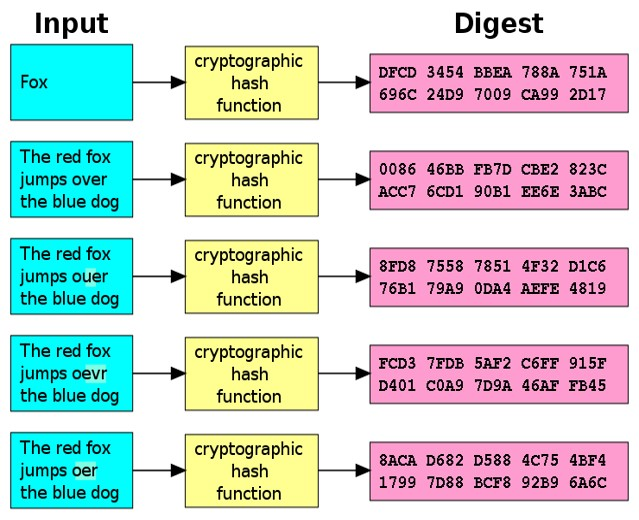# Cryptographic Hash Functions and Collisions

Different input messages are expected to produce different output hash values (message digest).## Hash Collisions

A collision means the same hash value for two different inputs. For simple hash functions it is easy to reach a collision. For example, assume a hash function h(text) sums of all character codes in a text. It will produce the same hash value (collision) for texts holding the same letters in different order, i.e. h('abc') == h('cab') == h('bca'). To avoid collisions, cryptographers have designed collision-resistant hash functions.

## Cryptographic Hash Functions: No Collisions

Collisions in the cryptographic hash functions are extremely unlikely to be found, so crypto hashes are considered to almost uniquely identify their corresponding input. Moreover, it is extremely hard to find an input message that hashes to given value.

Cryptographic hash functions are one-way hash functions, which are infeasible to invert. The chance to find a collision (by brute force) for a strong cryptographic hash function (like SHA-256) is extremely little. Let's define this in more details:

• Let's have hash value h=hash(p) for certain strong cryptographic hash function hash.
• It is expected to be extremely hard to find an input p', such that hash(p')=h.
• For most modern strong cryptographic hash functions there are no known collisions.

The ideal cryptographic hash function should have the following properties:

• Deterministic: the same input message should always result in the same hash value.
• Quick: it should be fast to compute the hash value for any given message.
• Hard to analyze: a small change to the input message should totally change the output hash value.
• Irreversible: generating a valid input message from its hash value should be infeasible. This means that there should be no significantly better way than brute force (try all possible input messages).
• No collisions: it should be extremely hard (or practically impossible) to find two different messages with the same hash.

Modern cryptographic hash functions (like SHA2 and SHA3) match the above properties and are used widely in cryptography.